Refer to our Texas Go Math Grade 3 Answer Key Pdf to score good marks in the exams. Test yourself by practicing the problems from Texas Go Math Grade 3 Lesson 5.4 Answer Key Model Addition and Subtraction.

Unlock the Problem

Sami scored 84 points in the first round of a new computer game. He scored 21 more points in the second round than in the first round. What was Sami’s total score?

You can use a strip diagram to solve the problem.

What do I need to find?
I need to find Sami’s total score.

What information am I given?
Sami scored 84 points in the first round.
He scored 21 more points than that in the second round.
Explanation:
Sami scored 84 points in the first round.
He scored 21 more points in the second round than in the first round.
Sami’s total score = 84 + 21 = 105

Plan

What is my plan or strategy?
I will draw a strip diagram to show the number of points Sami scored in each round.
Then I will use the strip diagram to decide which operation to use.

Solve

Complete the strip diagram to show the number of points Sami scored in the second round.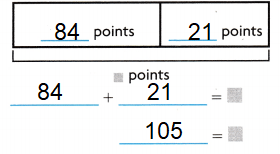Explanation:
Strip diagram is a rectangular model used to show numerical relationships.
It may be used to represent fractions, additions and subtractions also.

Complete another strip diagram to show Sami’s total score.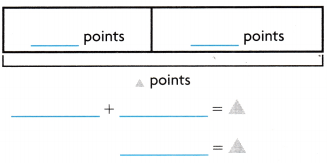Question 1.
How many points did Sami score in the second round?
21 points
Explanation:
It can be represented through strip diagram.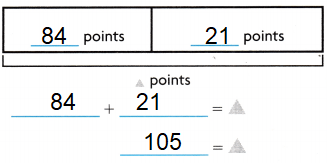What was Sami’s total score?
105 points
Explanation:
Total score can be found by using strip diagram as explained above.Try Another Problem

Anna scored 265 points in a computer game. Greg scored 142 points. How many more points did Anna score than Greg?

You can use a strip diagram model to solve the problem.

What do I need to find?
I need to find did Anna score more points than Greg.
Explanation:
From the above information we will come to know what to be found.

What information am I given?
Anna Score : 265
Greg Score : 142
Explanation:
The data is clearly given in the question.

Plan

What is my plan or strategy?
Strip diagram strategy.
Explanation:
I will draw a strip diagram to show the number of points Sami scored in each round.
Then I will use the strip diagram to decide which operation to use.

Solve

Record the steps you used to solve the problem.Strip record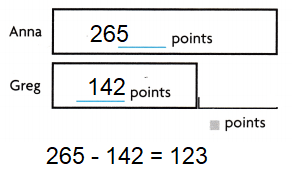Explanation:
Anna scored 265 points.
Greg scored 142 points.
Number of points did Anna score than Greg
265 – 142 = 123

Math Talk
Mathematical Processes

Explain how the length of each bar in the strip diagram would change if Greg scored more points than Anna but the totals remained the same.
The boxes will inter-change, and the total remains un change.
Explanation:
Anna scored 265 points.
Greg scored 142 points.
Number of points did Anna score than Greg
265 – 142 = 123How many more points did Anna score than Greg?
Explanation:
Anna scored 265 points.
Greg scored 142 points.
Number of points did Anna score than Greg
265 – 142 = 123Question 4.
When solving a math problem, we can check if the answer we have derived to is reasonable or not, based on an estimate.
A reasonable estimate does not exceed the original numbers in a problem.
Explanation:
Rounding the 265 to 260 and 142 to 140, the difference is 120
260 – 140 = 120
Since 123 is close to the estimate of 120, the answer is reasonable.

Question 5.
The strip drawing help us for solving the problem bigger the strip larger the number.
Explanation:
Strip diagram is a rectangular model used to show numerical relationships.
It may be used to represent fractions, additions and subtractions also.

Unlock the Problem
Use the Problem Solving Math Board.
Choose a strategy you know.

Share and Show

48 students voted
Explanation:
Number of students voted = 73 – 25 = 48 votes

Question 1.
Find how many students voted for Ben.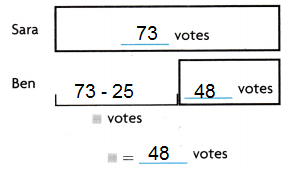Explanation:

Think: 73 – 25 = ☐
Write the numbers in the bars.
Find the total number of votes.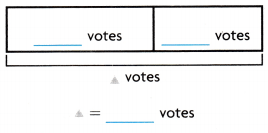Think: 73 + 48 = △
Write the numbers in the bars.
So, __121_______ students voted.
Explanation: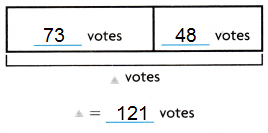Problem Solving

Question 2.
H.O.T. Multi-Step What if there were 3 students in another election and the total number of votes was the same? What would the strip diagram for the total number of votes look like? How many votes might each student get?Explanation: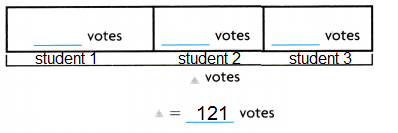H.O.T. Pose a Problem Use the strip diagram at the right. Write a problem to match it.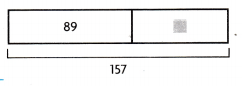Construction of word problem may vary.
For example;
In school election total votes polled were 157, out of which Sara received 89.How many voted did Ben received?
Explanation:
Ben received = 157 – 89 = 68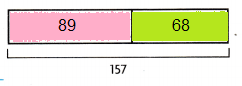Question 4.
Use Diagrams Solve the problem you wrote. Will you add or subtract?
SubtractExplanation:
Ben received = 157 – 89 = 68

Fill In the bubble for the correct answer choice. Use strip diagrams or equations to solve.

Question 5.
Caden drives 164 miles on the first two days of a business trip. He travels 77 miles on the third day. How many miles does he travel over three days?
(A) 87 miles
(B) 83 miles
(C) 231 miles
(D) 241 miles
Option (D)
Explanation:
Caden drives 164 first two days and third day 77 miles.
Total miles does he travel over three days.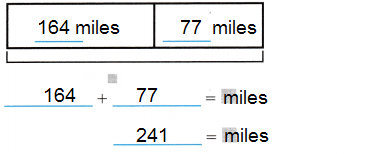Lauren reads 259 pages of a book in a week. David reads 183 pages in a week. How many more pages does Lauren read than David?
(A) 136
(B) 76
(C) 36
(D) 442
Option (B)
Explanation:
Lauren reads 259 pages of a book in a week.
David reads 183 pages in a week.
Number of pages does Lauren read than David.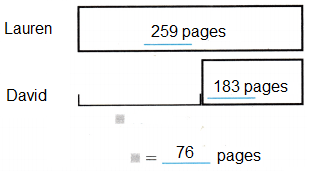Question 7.
Apply Multi-Step There are 306 people at the fair on Saturday. There are 124 fewer people on Sunday. How many people are at the fair on both Saturday and Sunday?
(A) 182
(B) 732
(C) 488
(D) 430
Option (C)
Explanation:
There are 306 people at the fair on Saturday.
There are 124 fewer people on Sunday.
Number of people are at the fair on both Saturday and Sunday.
306 – 124 =182
306 + 182 = 488Texas Test Prep

Question 8.
The number of laptop computers sold in one day was 42. That is 18 fewer than the number of desktop computers sold. How many desktop computers were sold?
(A) 61
(B) 50
(C) 60
(D) 24
Option (C)
Explanation:
The number of laptop computers sold in one day was 42.
That is 18 fewer than the number of desktop computers sold.
Total desktop computers were sold.
42 + 18 = 60

Texas Go Math Grade 3 Lesson 5.4 Homework and Practice Answer Key

Question 1.
There were 98 dogs and 47 cats at the pet show. How many fewer cats than dogs were at the show?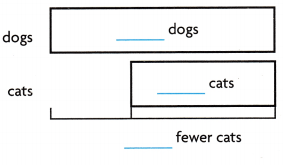51 dogs were at the show.
Explanation:
There were 98 dogs and 47 cats at the pet show.
Number of fewer cats than dogs were at the show = 98 – 47 = 51There were 56 parrots and 24 doves at the pet show. How many birds were at the pet show?80 birds at the pet show.
Explanation:
There were 56 parrots and 24 doves at the pet show.
Total birds at the pet showUse the strip diagram. Write a number sentence to match it.

Question 3.There are 107 live fishes in the shop tub, 24 were sold. how many fishes in the shop tub?
83 fishes in the shop tub.
Explanation:
There are 107 live fishes in the shop tub,
24 fishes were sold.
Total fishes left in the shop tub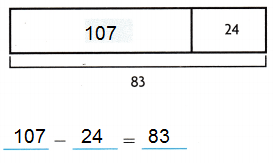Question 4.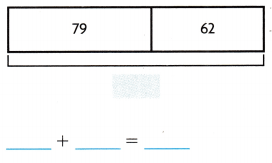David bought 79 pens for distributing in the Grade 3 class, he bought 62 again , now total how many pens David bought ?
David bought 136 pens.
Explanation:
David bought 79 pens for distributing in the Grade 3 class,
he bought 62 again ,
now total pens David bought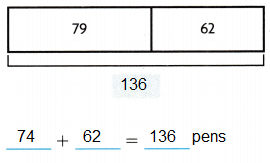Problem Solving

Question 5.
Marco goes bowling and scores 76 points in the first game. In the second game he scores 55 points. How many points does Marco score?
Marco scored 131 points.
Explanation:
Marco goes bowling and scores 76 points in the first game.
In the second game he scores 55 points.
Number of points does Marco score.Question 6.
Mrs. Mott picks 82 tomatoes in her garden. She picks 24 ears of corn. How many fewer ears of corn does she pick than tomatoes?
58 ears of corn.
Explanation:
Mrs. Mott picks 82 tomatoes in her garden.
She picks 24 ears of corn.
Total ears of corn does she pick than tomatoes.
82 – 24 = 58Lesson Check

Question 7.
Mr. Porter has 43 history books and 18 science books in his bookcase. How many books does Mr. Porter have?
(A) 58
(B) 14
(C) 51
(D) 61
Option (D)
Explanation:
Mr. Porter has 43 history books and 18 science books in his bookcase.
Total books does Mr. Porter have
43 + 18 = 61There are 347 students in the cafeteria. Of these, 123 are boys. How many of the students are girls?
(A) 247
(B) 470
(C) 324
(D) 224
Option (D)
Explanation:
There are 347 students in the cafeteria.
Of these, 123 are boys.
Number of girls
347 – 123 = 224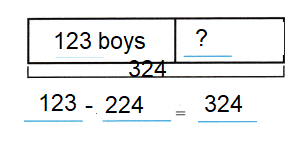Question 9.
Dwayne is reading a book that has 153 pages. He has read 77 pages. How many more pages does Dwayne have left to read?
(A) 220
(B) 76
(C) 230
(D) 86
Option (B)
Explanation:
Dwayne is reading a book that has 153 pages.
Number of pages does Dwayne have left to read
153 – 77 = 76Question 10.
Roxy counts 129 robins and 43 blue jays at her bird feeder. How many birds visit Roxy’s bird feeder?
(A) 172
(B) 162
(C) 86
(D) 96
Option (A)
Explanation:
Roxy counts 129 robins and 43 blue jays at her bird feeder.
Total birds visit Roxy’s bird feeder
129 + 43 = 172Question 11.
Multi-Step There are 208 people in one movie theater. There are 78 fewer people in the next movie theater. How many people are in both movie theaters?
(A) 286
(B) 338
(C) 130
(D) 296
Option (A)
Explanation:
There are 208 people in one movie theater.
There are 78 fewer people in the next movie theater.
The total number of people in both movie theaters
208 + 78 = 286

Go Math Practice and Homework Lesson 5.4 Answer Key Question 12.
Multi-Step Dan has 47 rocks in a collection. Milagros has 38 more rocks than Dan. How many rocks do Dan and Milagros have together in their rock collections?
(A) 85
(B) 132
(C) 75
(D) 122